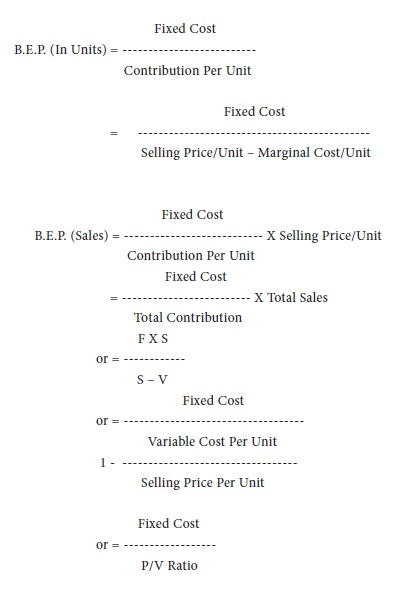Home | ARTS | Accounting For Managers | Break - Even or Cost-Volume-Profit Analysis - Marginal Costing

# Break - Even or Cost-Volume-Profit Analysis - Marginal Costing

Posted On :  26.02.2018 08:25 am

Break - Even or Cost-Volume-Profit Analysis

Break-even analysis is a specific method of presenting and studying the inner relationship between costs, volume and profits. (hence, the name c-v-p analysis). It is an important tool of financial analysis whereby the impact on profit of the changes in volume, price, costs and mix can be found out with a certain amount of accuracy. A business is said to break even when its total sales are equal to its total costs. It is a point of no profit or no loss. At this point contribution is equal to fixed costs. Break-even point, can be calculated thus:At break-even point the desired profit is zero. Where the volume of output or sales is to be calculated so as to earn a desired amount of profit, the amount of desired profits has to be added to the fixed cost given in the above formula.

Fixed Cost + Desired Profit
Units To Earn A Desired Profit = -------------------------------------
Contribution Per Unit

Fixed Cost + Desired Profit
Sales To Earn A Desired Profit = ------------------------------------
P/V Ratio

Tags : Accounting For Managers - Management Accounting-Marginal Costing
Last 30 days 1264 views